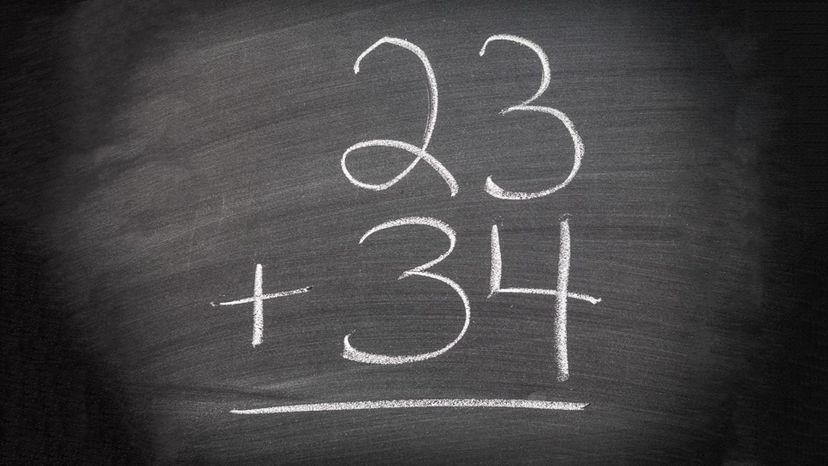# Can You Pass This Basic 3-Digit Number Addition Test Without Using a Calculator?

By: Zoe SamuelImage: Shutterstock

Remember how we all used to say, "But Miss Mathteacher, we can just use a calculator?" and she would put us in our places by saying, "You won't always have one with you when you're out and about, will you?" Then along came the smartphone, with its dozens of apps, the least of which can calculate far more with a few taps than even Stephen Hawking could do in his head. Oh, how we laughed at Miss Mathteacher! What a fool she was, to be overtaken by technology - and so quickly!

That's why, doubtless, you've kept that part of your brain spick and span ... and now's your chance to prove it!

What is 666 + 583?
1240
1249
1230
1250

What is 2.02 + 2.12?
2.22
4.14
That's right, if you have a 2 and another 2, the answer has to be over 4. You don't even need to look after the decimals.
2.00
2.21

What is 243 + 838?
900
1099
999
1081

What is 644 + 644?
1200
1150
1080
1288

What is 19.3 + 833?
852.3
Oh, those cheeky decimals! Here they are working together with an integer to mess with your head.
834
845
852

What is 334 + 924?
777
737
1258
1499

What is 833 + 226?
942
958
1059
998

What is 728 + 147?
122
797
875
An odd and an even? That's an odd.
804

What is 737 + 222?
959
550
990
840

What is 333 + 232?
799
565
787
698

What is 727 + 737?
1460
1400
1500
1464

What is 484 + 0.01?
494
484.01
We're down into the hundredths. Technically, this is still a triple-digit number, it's just much smaller than some.
474
484

What is 833 + 484?
1316
1318
1320
1317
An odd and an even number always make an odd number.

What is 501 + 499?
900
1000
The right answer is 1000 flat.
600
1100

What is 273 + 964?
1022
1237
988
1050

What is 2.33 + 10.1?
11.43
12.43
One of these is to one decimal place, the other to two. That's confusing unless you are on the ball.
15.43
20.43

What is 172 + 182?
273
354
220
351

What is 100 + 1.00?
200
101
We fooled you with the decimal ... or did we? That's right, we're getting into decimals now.
500
1000

What is 10.4 + 3.22?
130.2
132.6
13.62
132.6

What is 353 + 111?
464
564
664
764

What is -284 + 101?
-183
Even though some progress was made back towards 0, it's still negative!
283
-384
-484

What is 836 + 183?
1019
1001
999
1011

What is 9.24 + 82.3?
101.1
82.34
91.54
This looks like it should end in an odd number but actually it doesn't have to. That's because .3 is really .30.
76.01

What is 743 + 199?
200
942
1042
943

What is 5.43 + 300?
503.40
305.43
It's an integer plus a decimal, so of course it can't be a round number. You just have to think of the integer as having .00 after it.
350.40
403.55

What is 721 + 279?
1200
1150
1000
It's 1000 again.
950

What is 3.12 + 1.09?
40.2
42.1
4.21
They're little numbers so the answer is little!
40.1

What is 4.53 + 9.99?
14.52
More tricky decimals! But here it's still a fairly simple business to add them up.
1.52
99.43
103.4

What is 294 + 6.00?
310
299.5
300
300 is a round number and anything ending .00 is a round number.
301.05

What is 373 + 211?
584
699
564
400

What is 991+101?
1092
1022
1011
1024

What is -100 + -101?
-201
Did you notice the negative signs? If you did, you'll have gotten the right answer.
200
201
2001

What is -712 + -274?
548
-986
Two negatives, so you just go all the way negative!
-548
-106

What is -342 + 733?
1075
733
391
There's one positive and one negative number here, so you just deduct the negative from the positive.
-391

What is -377 + -223?
1000
600
-154
-600
It's two negative numbers here. So you just add them together and put a minus sign in front of it!